# Test: Single Correct MCQs: Sequences and Series | JEE Advanced

## 19 Questions MCQ Test Mathematics For JEE | Test: Single Correct MCQs: Sequences and Series | JEE Advanced

Description
Attempt Test: Single Correct MCQs: Sequences and Series | JEE Advanced | 19 questions in 40 minutes | Mock test for JEE preparation | Free important questions MCQ to study Mathematics For JEE for JEE Exam | Download free PDF with solutions
QUESTION: 1

### If x, y and z are pth, qth and rth terms respectively of an A.P. and also of a G.P., then xy – z yz – x zx – y is equal to :

Solution:

∵ x, y, z are the pth, qth and rth terms of an  AP..
∴ x = A + (p – 1)D; y = A + (q – 1) D; z  = A + (r – 1) D
⇒ x – y = (p – q)D;  y – z = (q – r) D z – x = (r – p) D ....(1)
where A is the first term and D is the common difference.
Also x, y, z are the pth, qth, and rth terms of a GP.
∴ x = ARp – 1, y = ARq – 1, z = ARr – 1
∴ xy – z yz – x zx –y = (ARp –1)y–z (ARq–1)z–x (ARr–1)x–y
= Ay–z+z–x+x–yR(p–1)(y–z)+(q–1)(z–x)+(r–1)(x–y)
= A0r(p–1) (q–r) D+(q–1)(r–p)D+(r–1)(p–q)D = A0R0 =1

QUESTION: 2

### The third term of a geometric progression is 4. The product of the first five terms is (1982 - 2 Marks)

Solution:

ar2 = 4 ....(1)

a. ar. ar2 .ar3 .ar4 = a5 r10 = (ar2)5 = 45.

QUESTION: 3

### The rational  number, which equals the number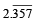with recurring decimal is (1983 - 1 Mark)

Solution: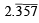= 2 + .357 + 0.000357 + ...∞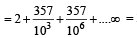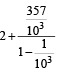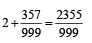QUESTION: 4

If a, b, c are in G.P., then the equations ax 2 + 2bx + c = 0 and dx 2 + 2ex + f = 0 have a common root if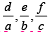are in –– (1985 - 2 Marks)

Solution:

a, b, c are in G.P. b2 = ac ....(1)

ax2  + 2bx + c = 0 and dx2  + 2ex + f = 0 have a common root

Let it be α, then aα2 + 2bα + c = 0 dα2 + 2eα + f = 0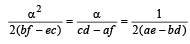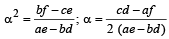Substituting the value of α , we get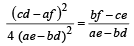⇒ (cd – af) 2 = 4 (ae – bd) (bf – ce)

Dividing both sides by a2c2 we get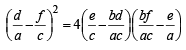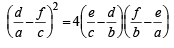[Using eq. (1)]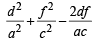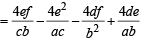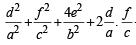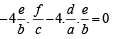[Using eq.(1)]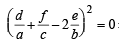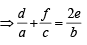⇒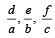are in A.P..

QUESTION: 5

Sum of the first n terms of the series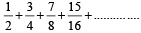....... is equal to (1988 - 2 Marks)

Solution:

Let S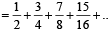n terms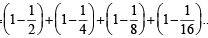..n terms

= (1 + 1 + 1 + ....n  terms)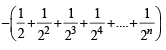=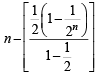= n - 1+2-n

QUESTION: 6

The number log 2 7 is (1990 -  2 Marks)

Solution:

We know that log2 4 = 2 and log2 8 = 3
∴ log2 7 lies between 2 and 3
∴ log2 7 is either rational or irrational but not integer or prime number.
If possible let log2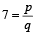(a rational number)

⇒ 2p/q = 7
⇒ 2p = 7q
⇒ even number = odd number
∴ We get a contradiction, so assumption is wrong.
Hence log2 7 must be an irrational number.

QUESTION: 7

If ln(a + c), ln (a – c), ln (a – 2b + c) are in A.P., then  (1994)

Solution:

In (a + c), In (a – c), In (a – 2b + c) are in A.P.
⇒ a + c, a – c, a – 2b + c are in G.P.
⇒ (c – a)2 = (a + c) (a –2b + c)
⇒ (c – a)2 = (a + c)2 – 2b (a + c)
⇒ 2b (a + c) = (a + c)2 – (c – a)2
⇒ 2b (a + c) = 4ac ⇒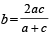⇒ a, b, c  are in H.P

QUESTION: 8

Let a1, a2, ..... a10 be in A, P, and h1, h2,....h10 be in H.P. If a1 = h1 = 2 and a10 = h10 = 3, then a4h7 is (1999 - 2 Marks)

Solution:

a1= h1= 2, a10 = h10 = 3
3 = a10 = 2 + 9d ⇒ d = 1/9
∴ a4 = 2 + 3d = 7/3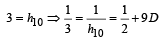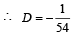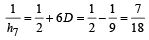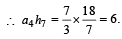QUESTION: 9

The harmonic mean of the roots of the equation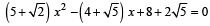is (1999 - 2 Marks)

Solution: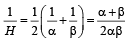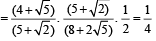∴ H = 4.

QUESTION: 10

Consider an infinite geometric series with first term a and common ratio r. If its sum is 4 and the second term is 3/4, then                 (2000S)

Solution:

Sum = 4 and second term = 3/4, it is given that first term is a and common ratio r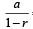= 4 and ar = 3 /4 ⇒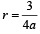Therefore,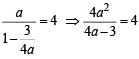or a2 – 4a + 3 = 0 ⇒ (a – 1)  (a – 3) = 0
⇒ a = 1  or  3
When a = 1, r = 3/4 and when a = 3, r = 1/4

QUESTION: 11

Let α, β be the roots of x2 - x + p = 0 and γ, δ be the roots of x2 - 4x + q = 0. If α, β, γ, δ are in G.P., then the integral values of p and q respectively, are (2001S)

Solution:

α, β are the roots of x2 – x + p=0
∴ α + β = 1 ....(1)
αβ = p ...(2)
g,δ are the roots of x2 – 4x  + q = 0

∴ γ + δ = 4....(3)
γδ = q ....(4)
α,β, γ,δ are in G.P..
∴   Let α = a;  β = ar, γ = ar 2 , δ = ar3 .
Substituting these values in equations (1), (2), (3) and (4), we get

a + ar = 1 ....(5)
a2r = p ....(6)
ar2 + ar3 = 4 ....(7)
a2r5 = q ....(8)
Dividing (7)  by  (5)  we get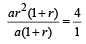⇒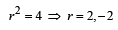(5) ⇒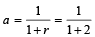or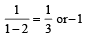As p is an integer (given), r is also an integer (2 or – 2).

∴ (6) ⇒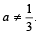Hence a = -1 and r = -2.

∴ p = (– 1)2 × (– 2) = – 2

q = (– 1)2 × (– 2)5 = – 32

QUESTION: 12

Let the positive numbers a, b, c, d be in A.P. Then abc, abd, acd, bcd are (2001S)

Solution:

a, b, c, d are in A.P.

∴ d, c, b, a are also in A.P.

⇒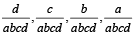are also in A.P..

⇒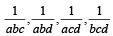are in A.P..

⇒ abc, abd, acd, bcd are in H.P.

QUESTION: 13

If the sum of the first 2n terms of the A.P. 2, 5, 8, …, is equal to the sum of the first n terms of the A.P. 57, 59, 61, …, then n equals(2001S)

Solution:

ATQ  2 + 5 + 8 + .... 2n terms = 57 + 59 + 61 + .... n terms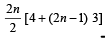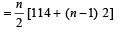⇒ 6n + 1= n + 56 ⇒ 5n = 55 ⇒ n = 11

QUESTION: 14

Suppose a, b, c are in A.P. and a2, b2, c2 are in G.P. if a < b < c and a + b + c =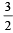, then the value of a is (2002S)

Solution:

Given that a, b, c are in A.P.
⇒ 2b = a + c
⇒ but given a + b + c = 3/2
⇒  3b = 3/2
⇒ b = 1/2 and then a + c = 1
Again a2, b2, c2, are in G.P. ⇒ b4 = a2 c2

⇒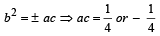and a + c = 1 ....(1) Considering a + c = 1 and ac = 1/4
⇒ (a – c)2 = 1 –  1 = 0   ⇒ a = c
but a ≠ c as given that a < b < c
∴ We consider a + c = 1 and ac = – 1/4
⇒ (a – c)2 = 1 + 1= 2 ⇒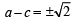but a < c ⇒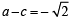....(2)

Solving (1) and (2) we get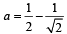QUESTION: 15

An infinite G.P. has first term ‘x’ and sum ‘5’, then x belongs to    (2004S)

Solution: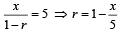Since G.P. contains infinite terms

∴ – 1 < r < 1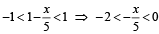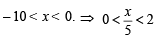⇒ 0 < x< 10.

QUESTION: 16

In the quadratic equation ax2 + bx + c = 0, Δ  = b2  – 4ac and α + β, α2 + β2, α3 + β3, are in G.P. where a, b are the root of ax2 + bx + c = 0, then (2005S)

Solution:

In the quadratic equation ax2 + bx + c = 0

Δ = b2 - 4ac and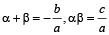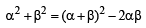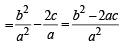and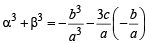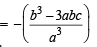Given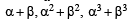are in G.P.

⇒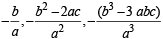are in G.P.

⇒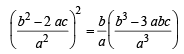⇒ b4 + 4a2c2 – 4ab2c = b4 – 3ab2c

⇒ 4a2c2 – ab2c = 0 ⇒ ac Δ = 0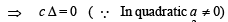QUESTION: 17

In the sum of first n terms of an A.P. is cn2, then the sum of squares of these n  terms is (2009)

Solution:

Given that for an A.P.Sn = cn2

Then Tn = Sn-Sn-1 = cn2 - c(n- 1)2 = (2n- 1)c

∴ Sum of squares of n terms of this A.P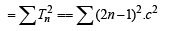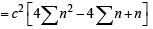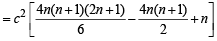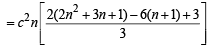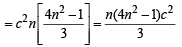QUESTION: 18

Let a1, a2, a3, ..... be in harmonic progression with a1 = 5 and a20 = 25. The least positive integer n for which an < 0 is    (2012 )

Solution:

∵ a1, a2, a3, ....... are in H.P..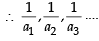.. are in A.P..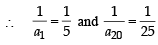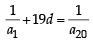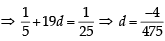Now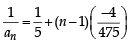Clearly  an < 0  if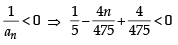⇒ – 4n < – 99   or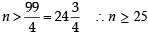Hence least value of n is 25.

QUESTION: 19

Let bi > 1 for i = 1, 2, ..., 101. Suppose loge b1,  loge b2, ...., loge b101 are in Arithmetic Progression (A.P.) with the common difference loge 2. Suppose a1, a2, ...., a101 are in A.P. such that a1= b1 and a51= b51. If t= b1+b2 + .... + b51 and s= a1+a2+ .... + a53, then   (JEE Adv. 2016)

Solution:

logc b1, logc b2,----, logcb101 are in A.P.
⇒ b1, b2,---- b101 are in G.P.
Also a1, a2,---- a101 are in A.P. where a1 = b1 are a51 = b51.
∴ b2, b3,---,b50 an d GM’s and a2, a3,----, a50 are AM’s between b1 and b51.
∵ GM < AM ⇒ b2 < a2, b3 < a3,----, b50 < a50
∵ b1 + b2 +----+ b51 <  a1 + a2 +----+ a51
⇒ t < s Also a1, a51, a101 are in AP b1, b51, b101 are in GP
∵ a1 = b1 and a51 = b51
∴ b101 > a101Use Code STAYHOME200 and get INR 200 additional OFF Use Coupon Code